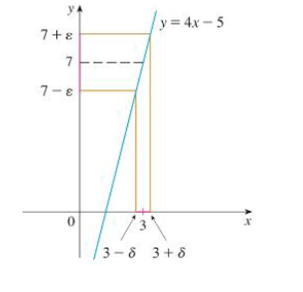Chapter 2.4, Problem 16E

Chapter
Section
Textbook Problem

Prove the statement using the ε, δ definition of a limit and illustrate with a diagram like Figure 9.FIGURE9lim x → 4 ( 2 x − 5 ) = 3

To determine

To prove: The statement limx4(2x5)=3.

Explanation

Definition used:

“Let f be a function defined on some open interval that contains the number a, except possibly at a itself. Then, the limit of f(x) as x approaches a is L.

That is, limxaf(x)=L if for every number ε>0 there is a number δ>0 such that if 0<|xa|<δ then |f(x)L|<ε”.

Let ε be a given positive integer. Here, a=4, L=3 and f(x)=2x5.

By the definition of ε and δ, it is enough to find a number δ such that, if 0<|x4|<δ, then |(2x5)3|<ε.

Consider |(2x5)3|.

|(2x5)3|=|2x53|=|2x8|=|2||x4|=2|x4|

Therefore, if 0<|x4|<δ, then 2|x4|<ε.

That is, if 0<|x4|<δ, then |x4|<ε2

Still sussing out bartleby?

Check out a sample textbook solution.

See a sample solution

The Solution to Your Study Problems

Bartleby provides explanations to thousands of textbook problems written by our experts, many with advanced degrees!

Get Started

Expand each expression in Exercises 122. (2x2)(3x4)

Finite Mathematics and Applied Calculus (MindTap Course List)

x+1x1=5

Applied Calculus for the Managerial, Life, and Social Sciences: A Brief Approach

Evaluate the integral. dxx(1+x)

Calculus (MindTap Course List)

Limits at Infinity Find the limit. 16. limx(3x3+x2)

Precalculus: Mathematics for Calculus (Standalone Book)

Finding a Limit In Exercises 1128, find the limit. limx04+x2x

Calculus: Early Transcendental Functions (MindTap Course List)

The graph of x = cos t, y = sin2 t is:

Study Guide for Stewart's Single Variable Calculus: Early Transcendentals, 8th